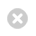Close

## UCL Mechanical Engineering Faculty of Engineering Sciences

Home# MECH0003 Introduction to Mechanical Engineering

## MECH0003 Introduction to Mechanical Engineering

FHEQ Level:
Term:
Term 1
UCL / ECTS Credits:
15 UCL / 7.5 ECTS
Previous Module Code:
MECH101P
Prerequisites:
None

## Taught By

Dr Manish Tiwari (Module Coordinator):
Manish Tiwari
Dr Paul Fromme:
Paul Fromme

## Module Overview

This module provides an introduction to Mechanical Engineering, covering fundamental concepts of Applied Mechanics (Statics) and Introduction to Thermofluids.

The Statics part will aim to teach the basic analytical methods, that is, the fundamental concepts and techniques of engineering mechanics (Statics). Building on mathematical skills from A-levels Mathematics (including, for some students, Mechanics modules) and the concurrent first year Mathematics module, basic concepts of Statics are introduced, practiced and applied to simple engineering problems. Students will obtain modelling knowledge, tools and experience appropriate for a first year engineering module, providing the foundation for higher level modules.

The second part of the module aims to teach fundamentals of thermofluid sciences. Building on the mathematical skills and physics learning from the A-levels and the concurrent first year Mathematics module, the basic concepts of control volume and control mass are introduced – this teaches students how to analyse systems with and without flows. These fundamental features are then used to perform mass, momentum and energy balance – both in isothermal and non-isothermal systems – with various levels of assumptions. The energy balance is introduced via the first law of Thermodynamics and solution of several analytical problems drawn from practical engineering applications.

## Topics Covered

Applied Mechanics – Statics

• Forces and moments
• Rigid body equilibrium
• Friction
• Analysis of structures
• Distributed forces and centre of gravity
• Internal forces and moments in structures

Introduction to Thermofluids

• Introduction to thermodynamics and related concepts of fluid mechanics
• Fundamental principles and properties of fluid media
• Analysing systems and devices
• Mass balance and energy in isothermal conditions
• Flow analysis
• Principle of energy conservation
• First Law of Thermodynamics with flow
• Application of First Law

## Learning Outcomes:

Upon completion of this module students will be able to:

• Demonstrate knowledge and understanding of the essential facts, concepts, theories and principles underlying fundamental fluid mechanics.
• Apply basic scientific principles of fluid mechanics to solve simple engineering problems involving modelling and analysis of basic engineering systems involving: simple flow systems, using appropriate conservation principles, and applying the principles of dimensional analysis and physical similarity to engineering model testing.
• Use pressure, temperature and flow measurement in dedicated test equipment and wind tunnels; analyse experimental results and draw conclusions, given specific guidance to the appropriate background material; estimate uncertainty of simple fluid mechanics and thermodynamics measurements.
• Apply a range of techniques to analyse available evidence and solve simple engineering problems pertaining to fluid flow systems.

## Method of Instruction

This module is taught through:

• Lectures
• Tutorials
• Practical laboratory classes

## Assessment

This module is assessed entirely through coursework exercises.